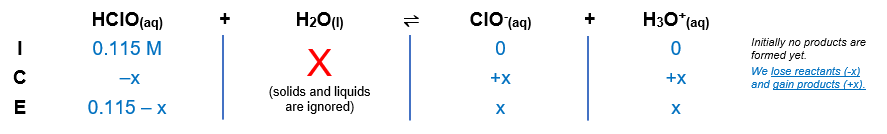# Problem: Hypochlorous acid, HClO, has a pKa of 7.54. What are [H3O+], pH, [ClO−], and [HClO] in 0.115 M HClO?

###### FREE Expert Solution

Equilibrium reaction:        HClO(aq) + H2O(l)  ClO-(aq) + H3O+(aq)

Step 1: Construct an ICE chart for the reaction.Step 2: Write the Ka expression and calculate for Ka.

${\mathbf{K}}_{{\mathbf{a}}}\mathbf{=}\frac{\mathbf{products}}{\mathbf{reactants}}\phantom{\rule{0ex}{0ex}}\overline{){\mathbf{K}}_{\mathbf{a}}\mathbf{=}\frac{\mathbf{\left[}{\mathbf{ClO}}^{\mathbf{-}}\mathbf{\right]}\mathbf{\left[}{\mathbf{H}}_{\mathbf{3}}{\mathbf{O}}^{\mathbf{+}}\mathbf{\right]}}{\left[\mathrm{HClO}\right]}}$

Solids and liquids are not included in the expression

${\mathbf{K}}_{\mathbf{a}}\mathbf{=}{\mathbf{10}}^{\mathbf{-}{\mathbf{pK}}_{\mathbf{a}}}\phantom{\rule{0ex}{0ex}}{\mathbf{K}}_{\mathbf{a}}\mathbf{=}{\mathbf{10}}^{\mathbf{-}\mathbf{7}\mathbf{.}\mathbf{54}}$

Ka = 2.884x10-8

Step 3: Calculate the equilibrium concentrations.

${\mathbf{K}}_{\mathbf{a}}\mathbf{=}\frac{\left[x\right]\left[x\right]}{\left[0.115-x\right]}\phantom{\rule{0ex}{0ex}}\mathbf{2}\mathbf{.}\mathbf{884}\mathbf{×}{\mathbf{10}}^{\mathbf{-}\mathbf{8}}\mathbf{=}\frac{{\mathbf{x}}^{\mathbf{2}}}{\mathbf{0}\mathbf{.}\mathbf{115}\mathbf{-}\mathbf{x}}$

$\mathbf{2}\mathbf{.}\mathbf{884}\mathbf{×}{\mathbf{10}}^{\mathbf{-}\mathbf{8}}\mathbf{\left(}\mathbf{0}\mathbf{.}\mathbf{115}\mathbf{\right)}\mathbf{=}\frac{{\mathbf{x}}^{\mathbf{2}}}{\overline{)\mathbf{0}\mathbf{.}\mathbf{115}}}\overline{)\left(0.115\right)}\phantom{\rule{0ex}{0ex}}\sqrt{\mathbf{3}\mathbf{.}\mathbf{3166}\mathbf{×}{\mathbf{10}}^{\mathbf{-}\mathbf{9}}}\mathbf{=}\sqrt{{\mathbf{x}}^{\mathbf{2}}}$

92% (154 ratings)###### Problem Details

Hypochlorous acid, HClO, has a pKa of 7.54. What are [H3O+], pH, [ClO], and [HClO] in 0.115 M HClO?